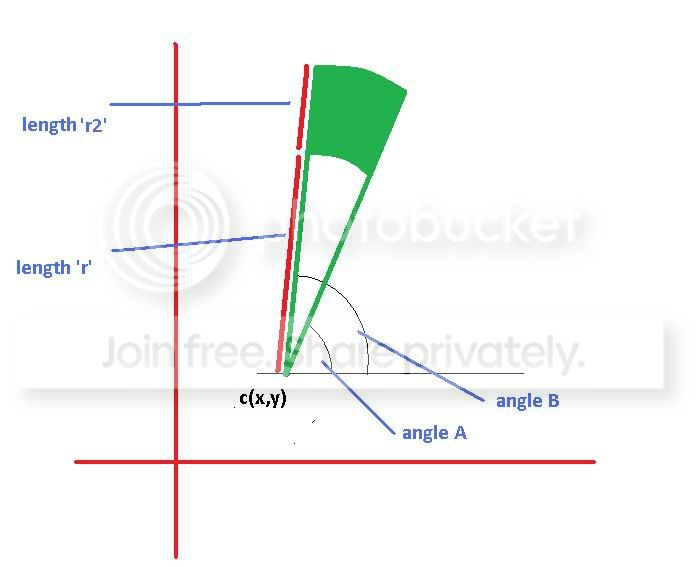# Calculating the bounding points for the area of a “Pie Segment” and “sub areas”

•
•  |
•

### Question

Background:

I was recently playing around with GDI+ to draw a "Disc" displaying a sweeping color change through 360 degrees. (I dug up some HSL to RGB code to loop through HSL(1,1,1) -> HSL(360,1,1))

Regarding the disc, I first drew a full solid circle using the above, and then a second circle in Grey over the center to give the followingSo this is all fine... but I realised that GDI+ is insulating us from a lot of the tricky match that's going on here by way of the `FillPie` method. Also, `FillPie` requires you to supply a bounding rectangle for the pie as opposed to a Radius Length. It also does a full segment fill and doesnt allow you to specify a part of that segment only.

Question:

Can anyone point me in the direction of some Math functions or give any explanation on what forumla I would need to calculate the area & plot points of the following "Green Filled Area" given:

``````Point `c` - an x,y co-ordinate
Angle `A` - an angle from horizontal
Angle `B  - an angle from horizontal where `B` - `A` == the sweep angle
Length `r` - a distance from `c`
Length `r2` - a distance from `c` where `r2` - `r` == the `height` of the segment to be filled.
``````Links to Math sources are fine but I've had a quick google & look at Wolfram Math and could find what I was looking for. Also, if there was some way to generate a sequence of bounding (x,y) co-or's that could be passed as a `Point[]` to Graphics.FillPolygon, that'd be cool too.

### Solution

The area is the difference of the outer and inner disc parts. The area of a disc part is proportional to the angle sweep:

``````area = (b-a)*((r+r2)^2-r^2)/2
``````

`a` and `b` must be expressed in radians. For `b-a = 2*Pi`, `area = Pi*(r+r2)^2 - Pi*r^2` is the difference of the areas of the outer and inner discs.

You can generate points on the inner / outer circle using

``````x = cx + r * cos(t)     /     x = cx + (r+r2) * cos(t)
y = cy + r * sin(t)     /     y = cy + (r+r2) * sin(t)
``````

Where `t` varies from `a` to `b`.

### OTHER TIPS

Hope this helps. The second part provides a method for calculating the area of a sector of a circle

http://www.wikihow.com/Calculate-the-Area-of-a-Circle

The area of a segment of a circle is simply the angle of the arc (in radians) times the radius. So the area of the green circle is obviously:

``````(B-A) * r2
``````

You need to draw lines (this pseudo code):

``````for aa from A to B
set color to required color // you could use aa in an equation with HSL to get something like your sample
x1=r*cos(aa)+x
y1=r*sin(aa)+y
x2=r1*cos(aa)+x
y2=r1*sin(aa)+y
draw line between (x1,y1) and (x2,y2)
``````

for a small-enough increment in the angles, and small-enough radii, this should be OK.

The points you're looking for are (x1,y1) and (x2,y2) for each angle aa